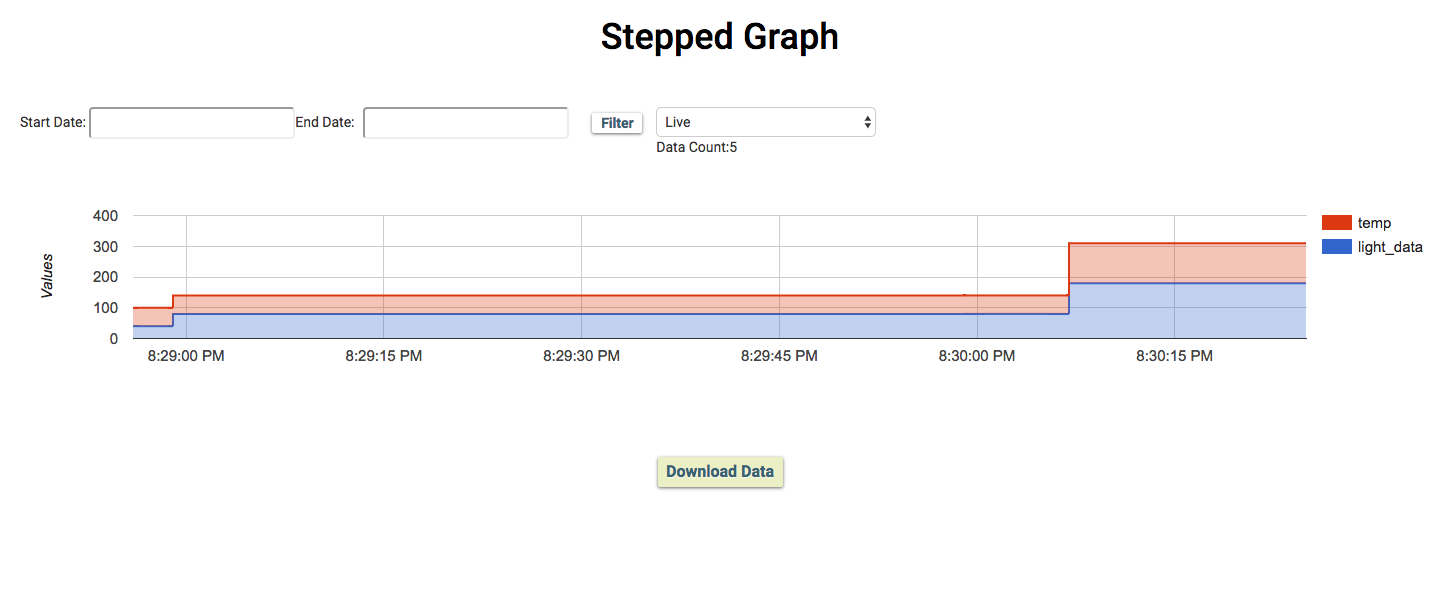# Stepped Graph

A Step chart is a Line chart that does not use the shortest distance to connect two data points. Instead, it uses vertical and horizontal lines to connect the data points in a series forming a step-like progression. The vertical parts of a Step chart denote changes in the data and their magnitude. The horizontal parts of a Step chart denote the constancy of the data.
Note: Choose the file extension as js.

# Sample Code

``````setChartLibrary('google-chart');
setChartType('steppedGraph');
setAxisName('time_stamp','light_data','temp');
plotChart('time_stamp','light_data','temp');
``````

# Output# Code Format

Syntax

Parameter(s)

Description

Library Name

Set the chart library to be used. Bolt Cloud uses 'google-chart' to visualize the data.

Graph Name

Set the title of the graph according to your choice.

setChartType('steppedGraph');

Graph type

Set the type of chart to be plotted. Bolt Cloud offers 8 different types of charts to be plotted i.e Line Graph, Bar Graph, Scatter Graph, Area Graph, Table Graph, Stepped Graph, Histogram Graph and Gauge.

setAxisName('time_stamp','first_variable_name','second_variable_name');

Name

Set the display name to differentiate between two different data sets.

plotChart('time_stamp','first_variable_name','second_variable_name');

Variable Names

Display the graph on the screen for the given variables.First parameter is always 'time_stamp' and other parameter is the variable names that you have given during hardware configuration of the product.

setNumberFormatter('format type');

Format type

Describes how numeric columns should be formatted. setNumberFormatter() function supports the following options as parameter - 'decimal', 'scientific', 'percentage', 'currencyInDollar', 'currencyInRupees'. This function should be called before the plotChart() function.

setDateFormatter('date format');

Date Format

Formats the x-axis date value in a variety of ways. setDateFormatter() function supports the following options as parameter- 'mm/dd/yyyy', 'dd/mm/yyyy'. This function should be called before the plotChart() function.